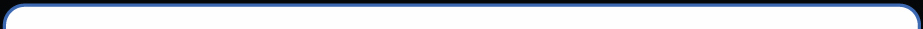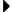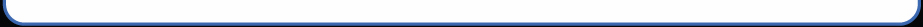Wire Gauge & Diameter Calculator (solid copper conductors) Enter Value: AWG Enter # of Conductors: Select Unit of Measurement: AWG inches millimeters mil square mil circular mil square millimetersHow to use this calculator... • Select a unit of measurement. AWG = American Wire Gauge. Inches, millimeters, and mils are for round wire diameter. • Enter the desired unit value the "Enter Value" box. • Enter the # of conductors to calculate for * Measurements are calculated based on the value of 1 wire. For example: If you choose Circular Mils and input 211600, it will assume a singular 4/0 AWG wire, not a bundle comprised of multiplef conductors.     < Back to Tech Room